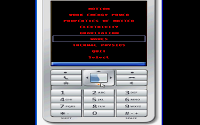Showing posts with label Physics. Show all posts
Showing posts with label Physics. Show all posts

### SI Ganaka

Seven Basic SI units are metre (Length), Kilogram (Mass), Second (Time), ampere (Electric Current) , Kelvin (Temperature), Candela (Luminous Intensity), Mole (Amount of Substance).  How do you calculate the values of Derived SI units values based on basic SI unit values. Remember all the formulas and do the calculations or use SI Ganaka. SI Ganaka calculates values of Derived SI units from Basic SI unit values.  Handy for Teachers in physics , chemistry or Maths and of course students. SI Ganaka  are useful to check at the values you arrive at doing the calculations manually.
SI Ganaka 1.2.1
SI Ganaka is a Derived SI units calculator.  The Basic  SI units are metre, kilogram, second, ampere, kelvin, candela, mole.  SI Ganaka calculates the Derived SI units values from Basic SI unit values. It calculates 49 derived unit values from Basic SI unit values.

It Calculates
1.AREA - Area, Volume, Specific Volume, Density, Concentration, Capacitance, Power,Radiant,Flux.
2.SPEED - Speed, Velocity, Acceleration, Force, Camel Train, Frequency, Pressure,Stress
3.THERMAL - Energy,Work,Quantity of Heat, Temperature in Celsius, Luminous Flux
4.ELECTRIC  - Electric Charge, Quantity of Electricity, Electric Potential, Potential Difference, Electromotive Force(EMF), Current Density,Capacitance
5.CONDUCTANCE - Electric Conductance, Electric Resistance, Inductance
6.MAGNETIC - Magnetic Field Strength, Magnetic Flux, Magnetic Flux Density.

Keycontrol
Up and Down Arrow keys to Browse Menu. Select or center key to select the Option. Touch screen choose the option and choose select. On the Parameter screen , you enter the data and select calculate. choose exit to get-back to menu. select quit to quit application.

Supporting Phones
All Java Enabled phone and Blackberry phones.
Both Touch and Non- Touch phones

### Physics Ganaka

Physics Ganaka
Calculators in Physics are becoming big part of physics learning, because unlike mathematics where we are only doing calculations, in Physics we need start with principles, theory and derivations and calculations. So a calculator comes in handy, especially for doing trivial ones. Physics Ganaka is an attempt at that. Physics are useful to check at the values you arrive at doing the calculations manually.

Physics Ganaka 1.2
Physics Ganaka is a Basic Physics calculator, Physics Ganaka calculates most of the fundamental calculations of fundamental physics.It Calculates
• Motion – Displacement, Momentum, Impulse, velocity,Force and also circular motion.
• Work, Kinetic Energy, Potential Energy, Power in Gravitational, Rotational and Elastic
• Matter – Density, Specific Gravity, Pressure, Specific Heat , Gas Laws
• Electricity – Current, Volt, Resistance, Power, Coulomb's Law
• Waves – Wave Speed, Wave length, Period, Frequency, Doppler Effect.
• Gravitation – Force, Acceleration, Potential Energy, Weight.
• Thermal – Specific Heat, Heat Transfer, ideal Gas Laws, Enthalpy, Helmholtz Energy
Keycontrol
Up and Down Arrow keys to Browse Menu. Select or center key to select the Option. Touch screen choose the option and choose select. On the Parameter screen , you enter the data and select calculate. choose exit to get-back to menu. select quit to quit application.

Supporting Phones
All Java Enabled phone and Blackberry phones.
Both Touch and Non- Touch phones